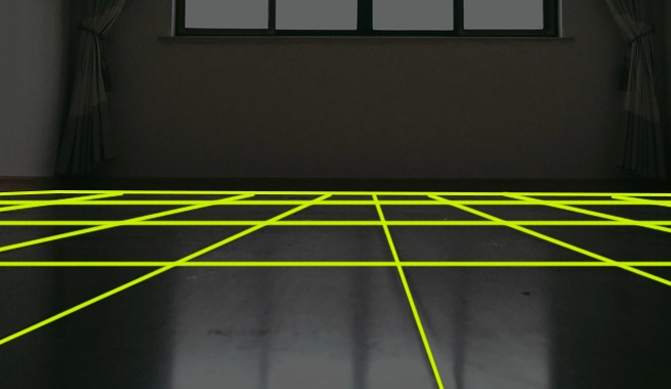# How to Measure Your Floor

Posted by We Sell Mats on

When shopping for floor mats, it’s important to have a good understanding of the area your mats need to cover, or, if you’re shopping for a gymnastics tumbling mat, whether your space is large enough to safely accommodate the mat you want. In this post we’ll deal with calculating square footages, so here’s a quick overview of how to measure your floor before purchasing interlocking floor mats.

## How Much Square Footage Do I Need?

Our EVA Foam Floor Mats are sold by square footage, so in order to know how much to order, you first need to measure your floor and calculate the square footage that you’ll need to cover. You may want to cover only a limited area of your floor, or you may want to do a wall-to-wall installation, so let’s cover both scenarios.

### Calculating a Limited Area

If you don’t need to trim your tiles to fit a specific space, simply use a tape measure to determine the length and width of the area where you want your mat to go, and then multiply the length x width to determine the square footage. For example, let’s say you wanted to add foam mats to one corner of your room:

Using your tape measure, you determine that an area of around 72 inches long and 48 inches wide will do the job. 72 x 48 = 3,456 square inches. To convert that to square feet, divide by 144. 3,456 ÷ 144 = 24 square feet. Now that you know how much area you’d like to cover, you can order a 24 square foot pack of your preferred interlocking tiles.

### Calculating a Wall-to-Wall Area

EVA Foam tiles are very easy to trim or cut, so even if your room dimensions are irregular or aren’t evenly divisible into 2-foot increments, you can still have a full wall-to-wall installation with careful measurement and trimming. Let’s take the same room as an example, but this time figure out how to determine the square footage required for a wall-to-wall installation. First, if you have some irregular spaces, imagine dividing the room into regular rectangles, and measure the length and width of each rectangle:

The three areas combined give you a total of 40,212 square inches. 40,212 ÷ 144 = 279.25 square feet. In any situation where you’re going to be cutting tiles, we strongly recommend purchasing at least 10% more coverage than your space actually demands. This will allow you some margin for error and also provide you with spare tiles should you ever need to replace one. That being the case, the minimum square footage we would recommend from among our pre-set packages is the 312 square foot pack. Keep reading to discover why more is better.

### How Many Tiles Do I Need?

You might wish to calculate the actual number of tiles you’ll need for your wall-to-wall installation. Since each 24" x 24" tile equals 4 square feet, you can get a rough estimate of the tile count by dividing your square footage by 4. In this case, 279.25 ÷ 4 = 69.8 tiles. To calculate the actual number of whole tiles you need, it helps to draw it out:

We’ve done the counting for you, and the actual number of tiles you’ll need for this wall-to-wall installation is 75. So even though the room is 279.25 square feet, you actually need 300 square feet of tiles in order to do the job, which is why we always recommend ordering at least 10% more coverage than your space requires. Even ordering the 312 square foot pack as described above would only give you 78 tiles, which is not a lot of room for error. Going for the 336 square foot pack would give you 84 tiles, and you’d have 9 extra tiles to account for mistakes in cutting or measuring.